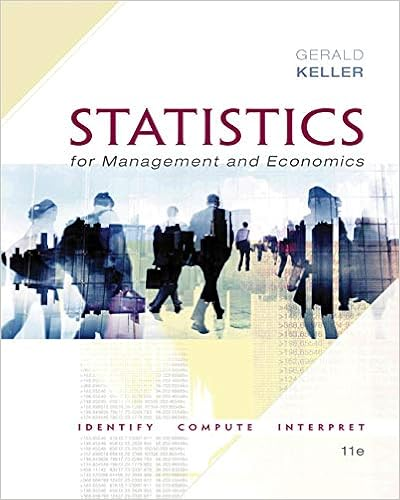# D identify the minimum and maximum values determine

• Lab Report
• 8

This preview shows page 2 - 5 out of 8 pages.

##### We have textbook solutions for you!
The document you are viewing contains questions related to this textbook.The document you are viewing contains questions related to this textbook.
Chapter 4 / Exercise 4.136
Statistics for Management and Economics + XLSTAT Bind-in
KellerExpert Verified
d. Identify the minimum and maximum values. Determine the Z-score of each. Do these values seem to be extreme outliers?
##### We have textbook solutions for you!
The document you are viewing contains questions related to this textbook.The document you are viewing contains questions related to this textbook.
Chapter 4 / Exercise 4.136
Statistics for Management and Economics + XLSTAT Bind-in
KellerExpert Verified
e. Compare the sample mean, median and standard deviation to the population values.
Central Limit Theorem for the Sample Mean (Questions 2,3,4) The lifetime of optical scanning drives follows a skewed distribution with µ =100 weeks and σ =100. The five columns labeled “CLT for mean n= ##” represent 1000 simulated random samples of 1, 5, 10, 30, and 100 from this population. 2. Make dot plots of all 5 sample sizes using the Multiple Y's Simple option and paste the result here. a. As the sample size changes, describe the change in center.
c. As the sample size changes, describe the change in shape.
•••